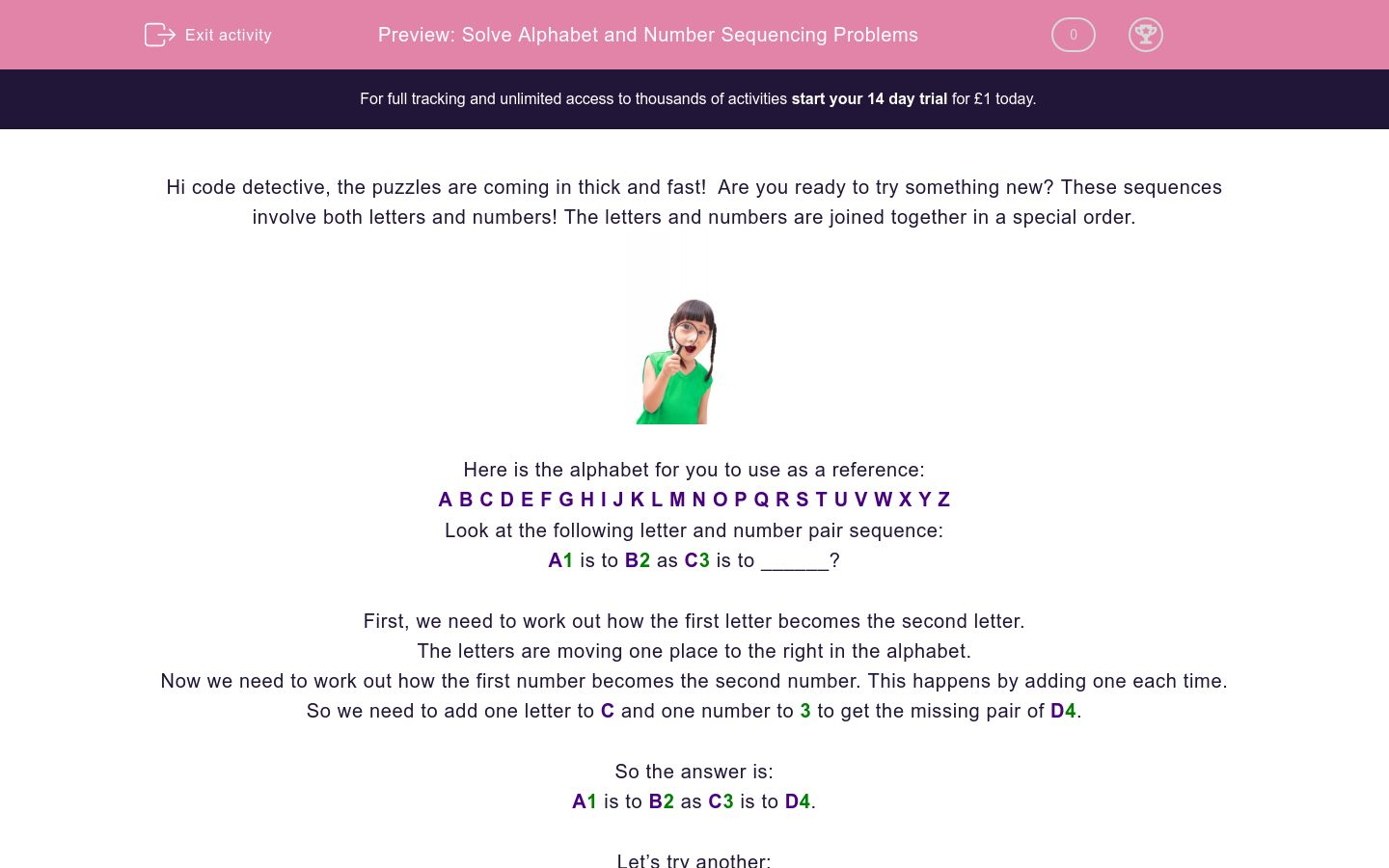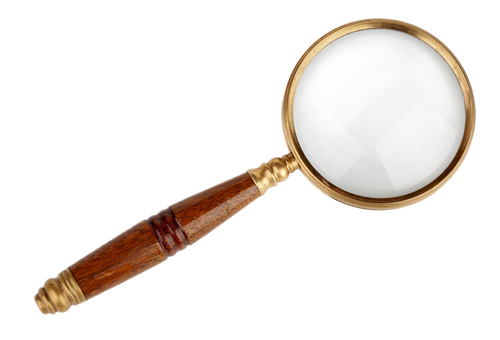# Solve Alphabet and Number Sequencing Problems

In this worksheet, students will solve simple sequences involving combined letter and number pairs. It will develop students’ problem solving and sequencing skills.Key stage:  KS 2

Curriculum topic:   Verbal Reasoning

Curriculum subtopic:   Letter Connections

Difficulty level:### QUESTION 1 of 10

Hi code detective, the puzzles are coming in thick and fast!  Are you ready to try something new? These sequences involve both letters and numbers! The letters and numbers are joined together in a special order.Here is the alphabet for you to use as a reference:

A B C D E F G H I J K L M N O P Q R S T U V W X Y Z

Look at the following letter and number pair sequence:

A1 is to B2 as C3 is to ______?

First, we need to work out how the first letter becomes the second letter.

The letters are moving one place to the right in the alphabet.

Now we need to work out how the first number becomes the second number. This happens by adding one each time.

So we need to add one letter to C and one number to 3 to get the missing pair of D4.

A1 is to B2 as C3 is to D4.

Let’s try another:

C4 is to E5 as F7 is to ______?

First, we need to work out how the first letter becomes the second letter.

The letters are moving two places to the right in the alphabet.

Now we need to work out how the first number becomes the second number. This happens by adding one each time.

So we need to add two letters to F and one number to 7 to get the missing pair of H8.

C4 is to E5 as F7 is to H8.

In this activity, you will need to be a sequence superstar to complete the number and letter pairs.

Remember to use the alphabet line to help you work out the code.

Here are two groups of letter and number sequences. Each group follows the same special sequence, but with different letters and numbers.

E6 is to F12 as H18 is to ______ ?A  B  C  D  E  F  G  H  I  J  K  L  M  N  O  P  Q  R  S  T  U  V  W  X  Y  Z

Here are two groups of letter and number sequences. Each group follows the same special sequence, but with different letters and numbers.

H5 is to N6 as Q42 is to ______ ?A  B  C  D  E  F  G  H  I  J  K  L  M  N  O  P  Q  R  S  T  U  V  W  X  Y  Z

Here are two groups of letter and number sequences. Each group follows the same special sequence, but with different letters and numbers.

G16 is to J18 as O20 is to ______ ?A  B  C  D  E  F  G  H  I  J  K  L  M  N  O  P  Q  R  S  T  U  V  W  X  Y  Z

Here are two groups of letter and number sequences. Each group follows the same special sequence, but with different letters and numbers.

C7 is to H10 as K20 is to ______ ?

 A  B  C  D  E  F  G  H  I  J  K  L  M  N  O  P  Q  R  S  T  U  V  W  X  Y  Z

Here are two groups of letter and number sequences. Each group follows the same special sequence, but with different letters and numbers.

Q23 is to U30 as L16 is to ______ ?A  B  C  D  E  F  G  H  I  J  K  L  M  N  O  P  Q  R  S  T  U  V  W  X  Y  Z

Here are two groups of numbers. Each group follows the same special sequence, but with different numbers.

O19 is to W24 as C13 is to ______ ?

 A  B  C  D  E  F  G  H  I  J  K  L  M  N  O  P  Q  R  S  T  U  V  W  X  Y  Z

Here are two groups of letter and number sequences. Each group follows the same special sequence, but with different letters and numbers.

C11 is to I19 as H21 is to ______ ?A  B  C  D  E  F  G  H  I  J  K  L  M  N  O  P  Q  R  S  T  U  V  W  X  Y  Z

Here are two groups of letter and number sequences. Each group follows the same special sequence, but with different letters and numbers.

E41 is to J45 as M32 is to ______ ?

 A  B  C  D  E  F  G  H  I  J  K  L  M  N  O  P  Q  R  S  T  U  V  W  X  Y  Z

Here are two groups of numbers. Each group follows the same special sequence, but with different numbers.

O29 is to U36 as M14 is to ______ ?

 A  B  C  D  E  F  G  H  I  J  K  L  M  N  O  P  Q  R  S  T  U  V  W  X  Y  Z

Here are two groups of letter and number sequences. Each group follows the same special sequence, but with different letters and numbers.

E59 is to N62 as C45 is to ______ ?A  B  C  D  E  F  G  H  I  J  K  L  M  N  O  P  Q  R  S  T  U  V  W  X  Y  Z

• Question 1

Here are two groups of letter and number sequences. Each group follows the same special sequence, but with different letters and numbers.

E6 is to F12 as H18 is to ______ ?A  B  C  D  E  F  G  H  I  J  K  L  M  N  O  P  Q  R  S  T  U  V  W  X  Y  Z
I24
EDDIE SAYS
Hi there, detective! There are two sequences here. The letter sequence follows the alphabet forwards one jump. The number sequence meanwhile, is add six.
• Question 2

Here are two groups of letter and number sequences. Each group follows the same special sequence, but with different letters and numbers.

H5 is to N6 as Q42 is to ______ ?A  B  C  D  E  F  G  H  I  J  K  L  M  N  O  P  Q  R  S  T  U  V  W  X  Y  Z
W43
EDDIE SAYS
The letter sequence is to make six jumps forwards in the alphabet. The number sequence is to add six. Great!
• Question 3

Here are two groups of letter and number sequences. Each group follows the same special sequence, but with different letters and numbers.

G16 is to J18 as O20 is to ______ ?A  B  C  D  E  F  G  H  I  J  K  L  M  N  O  P  Q  R  S  T  U  V  W  X  Y  Z
R22
EDDIE SAYS
Getting the hang of it? The letter sequence here is to take three jumps forwards in the alphabet. The number sequence is to add two.
• Question 4

Here are two groups of letter and number sequences. Each group follows the same special sequence, but with different letters and numbers.

C7 is to H10 as K20 is to ______ ?

 A  B  C  D  E  F  G  H  I  J  K  L  M  N  O  P  Q  R  S  T  U  V  W  X  Y  Z

P23
EDDIE SAYS
Brilliant effort! The letter sequence is to make five jumps forwards in the alphabet. The number sequence is to add three.
• Question 5

Here are two groups of letter and number sequences. Each group follows the same special sequence, but with different letters and numbers.

Q23 is to U30 as L16 is to ______ ?A  B  C  D  E  F  G  H  I  J  K  L  M  N  O  P  Q  R  S  T  U  V  W  X  Y  Z

P23
EDDIE SAYS
Did you find the missing letter and number? The letter sequence is to make four jumps forwards in the alphabet. The number sequence is to add seven.
• Question 6

Here are two groups of numbers. Each group follows the same special sequence, but with different numbers.

O19 is to W24 as C13 is to ______ ?

 A  B  C  D  E  F  G  H  I  J  K  L  M  N  O  P  Q  R  S  T  U  V  W  X  Y  Z
K18
EDDIE SAYS
Super sequencing skills! The letter sequence is to make eight jumps forwards in the alphabet. The number sequence is to add five.
• Question 7

Here are two groups of letter and number sequences. Each group follows the same special sequence, but with different letters and numbers.

C11 is to I19 as H21 is to ______ ?A  B  C  D  E  F  G  H  I  J  K  L  M  N  O  P  Q  R  S  T  U  V  W  X  Y  Z
N29
EDDIE SAYS
You're doing great, detective! The letter sequence is to make six jumps forwards in the alphabet. The number sequence is to add eight.
• Question 8

Here are two groups of letter and number sequences. Each group follows the same special sequence, but with different letters and numbers.

E41 is to J45 as M32 is to ______ ?

 A  B  C  D  E  F  G  H  I  J  K  L  M  N  O  P  Q  R  S  T  U  V  W  X  Y  Z

R36
EDDIE SAYS
Are you using the alphabet line to help you? The letter sequence here is to make five jumps forwards. The number sequence is to add four.
Whoosh, you're whizzing through these questions!
• Question 9

Here are two groups of numbers. Each group follows the same special sequence, but with different numbers.

O29 is to U36 as M14 is to ______ ?

 A  B  C  D  E  F  G  H  I  J  K  L  M  N  O  P  Q  R  S  T  U  V  W  X  Y  Z

S21
EDDIE SAYS
The letter sequence is to make six jumps forwards in the alphabet. The number sequence is to add seven. What a lot of jumping!
• Question 10

Here are two groups of letter and number sequences. Each group follows the same special sequence, but with different letters and numbers.

E59 is to N62 as C45 is to ______ ?A  B  C  D  E  F  G  H  I  J  K  L  M  N  O  P  Q  R  S  T  U  V  W  X  Y  Z

L48
EDDIE SAYS
The letter sequence is to make nine jumps forwards in the alphabet. The number sequence is to add three.
This super sequencing activity is now complete, hope to see you again soon, detective!
---- OR ----

Sign up for a £1 trial so you can track and measure your child's progress on this activity.

### What is EdPlace?

We're your National Curriculum aligned online education content provider helping each child succeed in English, maths and science from year 1 to GCSE. With an EdPlace account you’ll be able to track and measure progress, helping each child achieve their best. We build confidence and attainment by personalising each child’s learning at a level that suits them.

Get started